# Important Questions: Statistics

## 10 Questions MCQ Test NCERT Mathematics for CAT Preparation | Important Questions: Statistics

Description
Attempt Important Questions: Statistics | 10 questions in 10 minutes | Mock test for Class 10 preparation | Free important questions MCQ to study NCERT Mathematics for CAT Preparation for Class 10 Exam | Download free PDF with solutions
QUESTION: 1

Solution:
QUESTION: 2

### di is the deviation of xi from assumed mean a.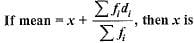Solution: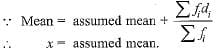QUESTION: 3

### Construction of a cumulative frequency table is useful in determining the

Solution:

A cumulative frequency table helps to determine the median of the series.

QUESTION: 4

Mean of 100 items is 49. It was discovered that three items which should have been 60, 70, 80 were wrongly read as 40, 20, 50 respectively. The correct mean is

Solution:

Sum of 100 observations = 100 x 49 = 4900
Correct sum = 4900 - [40 + 20 + 50] + [60 + 70 + 80] = 5000
∴ Correct mean = 5000/100 = 50

QUESTION: 5

In the formula, Mode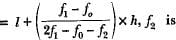Solution:
QUESTION: 6

Choose the correct answer from the given four options : In the formula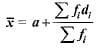for finding the mean of grouped data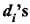are deviation from a of

Solution:
QUESTION: 7

For the following distribution: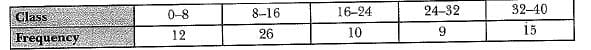The sum of upper limits of the median class and model class is

Solution:
QUESTION: 8

While computing mean of grouped data, we assume that the frequencies are

Solution:
QUESTION: 9

Consider the following frequency distribution of the height of 60 students of a class: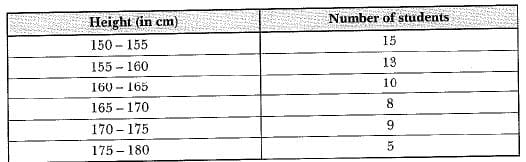The sum of the lower limit of the modal class and upper limit of the median class is

Solution:
QUESTION: 10

For the following distribution: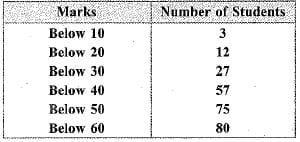the modal class is

Solution:Use Code STAYHOME200 and get INR 200 additional OFF Use Coupon Code